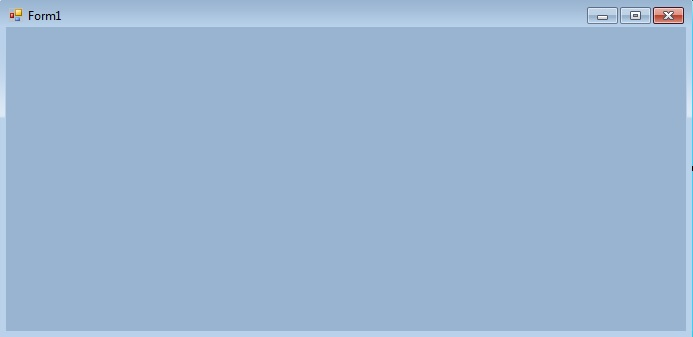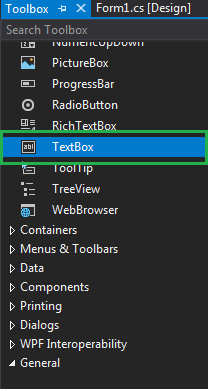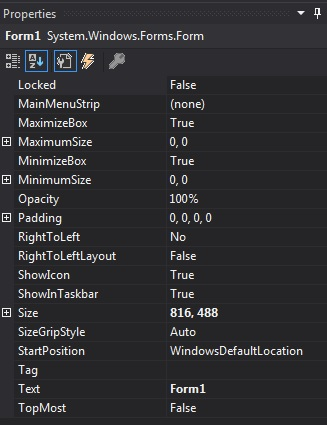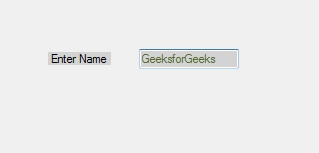GeeksforGeeks App
Open AppBrowser
Continue

In Windows forms, TextBox plays an important role. With the help of TextBox, the user can enter data in the application, it can be of a single line or of multiple lines. The TextBox is a class and it is defined under System.Windows.Forms namespace. In C#, you can create a TextBox in two different ways: 1. Design-Time: It is the simplest way to create a TextBox as shown in the following steps:

• Step 1: Create a windows form. As shown in the below image: Visual Studio -> File -> New -> Project -> WindowsFormApp• Step 2: Drag the TextBox control from the ToolBox and drop it on the windows form. You can place TextBox anywhere on the windows form according to your need.• Step 3: After drag and drop you will go to the properties of the TextBox control to modify the TextBox design according to your requirement.2. Run-Time: It is a little bit trickier than the above method. In this method, you can create your own textbox using the TextBox class.

• Step 1 : Create a textbox using the TextBox() constructor provided by the TextBox class.
```// Creating textbox
TextBox Mytextbox = new TextBox();```
• Step 2 : After creating TextBox, set the properties of the TextBox provided by the TextBox class.
```
// Set location of the textbox
Mytextbox.Location = new Point(187, 51);

// Set background color of the textbox
Mytextbox.BackColor = Color.LightGray;

// Set the foreground color of the textbox
Mytextbox.ForeColor = Color.DarkOliveGreen;

// Set the size of the textbox
Mytextbox.AutoSize = true;

// Set the name of the textbox
Mytextbox.Name = "text_box1";```
• Step 3 : And last add this textbox control to form using Add() method.
```// Add this textbox to form
 `using` `System;``using` `System.Collections.Generic;``using` `System.ComponentModel;``using` `System.Data;``using` `System.Drawing;``using` `System.Linq;``using` `System.Text;``using` `System.Threading.Tasks;``using` `System.Windows.Forms;` `namespace` `my {` `public` `partial` `class` `Form1 : Form {` `    ``public` `Form1()``    ``{``        ``InitializeComponent();``    ``}` `    ``private` `void` `Form1_Load(``object` `sender, EventArgs e)``    ``{` `        ``// Creating and setting the properties of Lable1``        ``Label Mylablel = ``new` `Label();``        ``Mylablel.Location = ``new` `Point(96, 54);``        ``Mylablel.Text = "Enter Name";``        ``Mylablel.AutoSize = ``true``;``        ``Mylablel.BackColor = Color.LightGray;` `        ``// Add this label to form``        ``this``.Controls.Add(Mylablel);` `        ``// Creating and setting the properties of TextBox1``        ``TextBox Mytextbox = ``new` `TextBox();``        ``Mytextbox.Location = ``new` `Point(187, 51);``        ``Mytextbox.BackColor = Color.LightGray;``        ``Mytextbox.ForeColor = Color.DarkOliveGreen;``        ``Mytextbox.AutoSize = ``true``;``        ``Mytextbox.Name = "text_box1";` `        ``// Add this textbox to form``        ``this``.Controls.Add(Mytextbox);``    ``}``}``}`
• Output: